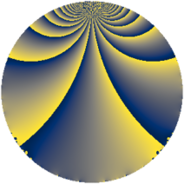# Properties

 Label 385.2.uLevel $385$ Weight $2$ Character orbit 385.u Rep. character $\chi_{385}(131,\cdot)$ Character field $\Q(\zeta_{6})$ Dimension $64$ Newform subspaces $1$ Sturm bound $96$ Trace bound $0$

# Related objects

## Defining parameters

 Level: $$N$$ $$=$$ $$385 = 5 \cdot 7 \cdot 11$$ Weight: $$k$$ $$=$$ $$2$$ Character orbit: $$[\chi]$$ $$=$$ 385.u (of order $$6$$ and degree $$2$$) Character conductor: $$\operatorname{cond}(\chi)$$ $$=$$ $$77$$ Character field: $$\Q(\zeta_{6})$$ Newform subspaces: $$1$$ Sturm bound: $$96$$ Trace bound: $$0$$

## Dimensions

The following table gives the dimensions of various subspaces of $$M_{2}(385, [\chi])$$.

Total New Old
Modular forms 104 64 40
Cusp forms 88 64 24
Eisenstein series 16 0 16

## Trace form

 $$64q + 28q^{4} + 36q^{9} + O(q^{10})$$ $$64q + 28q^{4} + 36q^{9} - 20q^{14} - 8q^{15} - 48q^{16} + 16q^{22} + 20q^{23} + 32q^{25} - 60q^{26} + 24q^{33} + 16q^{36} - 8q^{37} + 84q^{38} - 132q^{42} + 12q^{44} + 12q^{47} + 12q^{49} + 20q^{53} + 32q^{56} + 24q^{58} + 36q^{59} - 44q^{60} - 120q^{64} + 12q^{66} + 44q^{67} + 16q^{70} - 72q^{71} + 44q^{77} - 120q^{78} - 24q^{80} - 48q^{81} - 96q^{82} + 76q^{86} + 64q^{88} + 60q^{89} - 108q^{91} - 40q^{92} - 68q^{93} - 64q^{99} + O(q^{100})$$

## Decomposition of $$S_{2}^{\mathrm{new}}(385, [\chi])$$ into newform subspaces

Label Dim. $$A$$ Field CM Traces $q$-expansion
$$a_2$$ $$a_3$$ $$a_5$$ $$a_7$$
385.2.u.a $$64$$ $$3.074$$ None $$0$$ $$0$$ $$0$$ $$0$$

## Decomposition of $$S_{2}^{\mathrm{old}}(385, [\chi])$$ into lower level spaces

$$S_{2}^{\mathrm{old}}(385, [\chi]) \cong$$ $$S_{2}^{\mathrm{new}}(77, [\chi])$$$$^{\oplus 2}$$